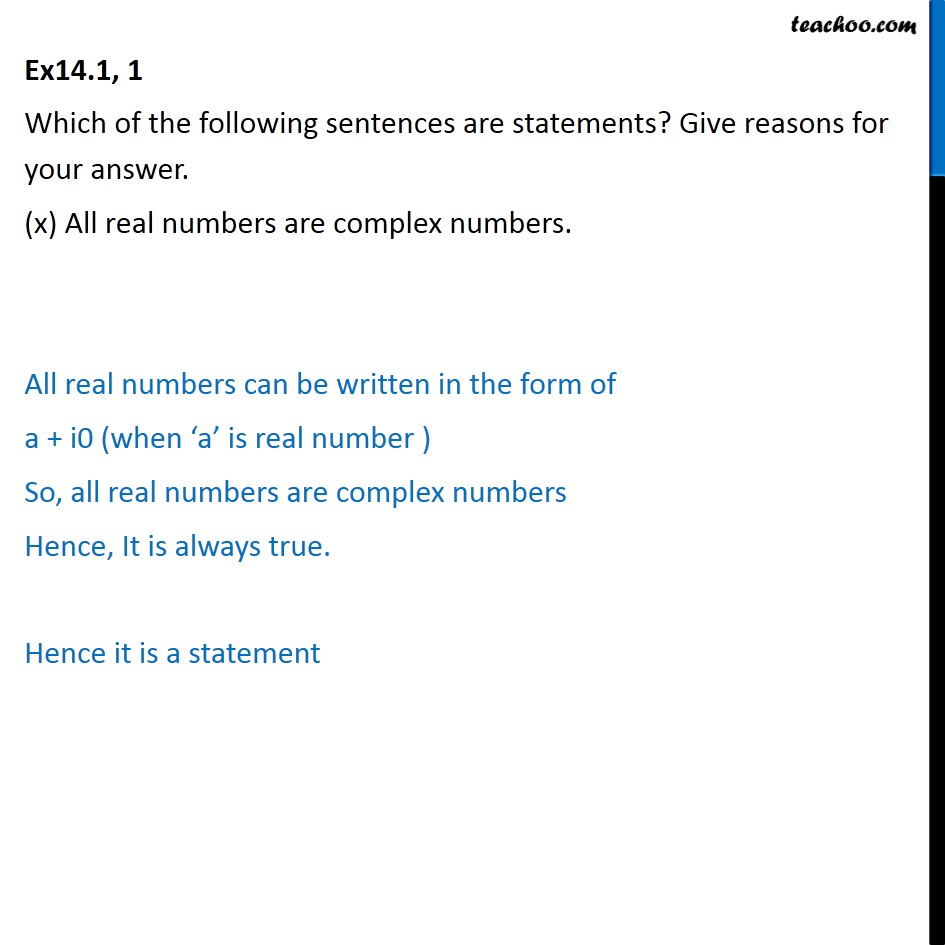Ex 14.1

Chapter 14 Class 11 Mathematical Reasoning
Serial order wiseMaths Crash Course - Live lectures + all videos + Real time Doubt solving!

### Transcript

Ex14.1, 1 Which of the following sentences are statements? Give reasons for your answer. (x) All real numbers are complex numbers. All real numbers can be written in the form of a + i0 (when ‘a’ is real number ) So, all real numbers are complex numbers Hence, It is always true. Hence it is a statement